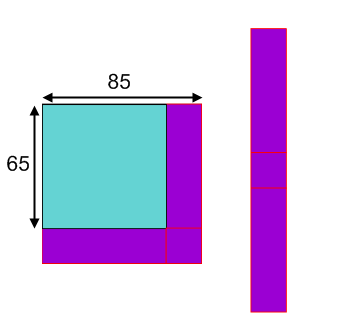#### You may also like### Euler's Squares

Euler found four whole numbers such that the sum of any two of the numbers is a perfect square...### DOTS Division

Take any pair of two digit numbers x=ab and y=cd where, without loss of generality, ab > cd . Form two 4 digit numbers r=abcd and s=cdab and calculate: {r^2 - s^2} /{x^2 - y^2}.### 2-digit Square

A 2-Digit number is squared. When this 2-digit number is reversed and squared, the difference between the squares is also a square. What is the 2-digit number?

# Plus Minus

### Why do this problem?

This problem offers students a chance to discover the identity for the difference of two square numbers. They are expected to work as research mathematicians, starting from simple examples, noticing patterns, developing conjectures and explanations that lead to proofs and further exploration.

It recognises the power of diagrams to help us make sense of algebraic generalisations.

### Possible approach

This problem follows on from Hollow Squares and/or What's Possible?

Write these first two examples from the problem on the board:
$$65^2-35^2=3000$$
$$55^2-45^2=1000$$
Ask students to suggest other pairs of numbers where the difference of their squares is a multiple of $1000$. Write their suggestions on the board.

If they only offer pairs of numbers that add up to $100$, write up
$$85^2-65^2=3000$$
$$85^2-35^2=6000$$

"Do you notice anything special about these pairs of numbers?"
Encourage students to use anything they notice to generate some more examples.

"Mathematicians are always interested in explaining anything that they notice."The following questions address the key ideas that we want students to encounter. Allow students plenty of time to reflect on these questions, maybe working in pairs initially, and discuss answers as a class:
"What is the connection between this diagram and the calculation $85^2-65^2$?"
"How could we work out the area of the long purple rectangle (without a calculator)?"
"Can you draw similar diagrams for some of our other calculations?"
"How can these diagrams help us to develop a quick method for evaluating $a^2-b^2$ for any values of $a$ and $b$?"

Now introduce students to another set of interesting results:
$$89^2-12^2=7777$$
$$78^2-23^2=5555$$
Can students explain why the digits are repeated, using the insights they have gained earlier?
Can they generate some similar examples?
How about $555555$? $434343$? $505505$?

When appropriate, bring the class together to share and compare strategies.
Finally, set students the task of exploring possibilities for generating "interesting" numbers of their own, to present to the rest of the class at the end.

Possible questions that students might wish to consider:

Can you write $1000, 2000, 3000...$ as the difference of two square numbers?
Can you write any of them in more than one way?
Can you write any repeated-digit number as the difference of two square numbers?
What about numbers like $123321$, $123456$...?

Another exploration that might yield conjectures about differences between square numbers is to look at the areas of rings of different widths. This article explains one student's work on this.

### Key questions

How does the diagram represent the difference between two square numbers?

How can the diagram be used to find a quick method for evaluating $a^2-b^2$ for any values of $a$ and $b$?

### Possible support

Pair Products is a good problem to introduce students to algebraic ways of representing general numerical results.

### Possible extension

Every odd prime number can be written as the difference of two squares. Prove that there is only one way to write an odd prime number as the difference of two squares, and that this is the difference of two successive squares.

120 can be written as the difference of the squares of whole numbers in the following ways: $$31^2-29^2$$
$$17^2-13^2$$
$$13^2-7^2$$
$$11^2-1^2$$

Can you find all the possible ways to write 924 as the difference of the squares of two whole numbers? Can you prove that you have found them all?

*REALLY CHALLENGING* Can you describe a way for working out how many ways there are to write any number as the difference of two perfect squares?

If I also allow myself to use decimals, I could write 120 as:
$$60.5^2-59.5^2$$
$$26.2^2-23.8^2$$
$$25.25^2-22.75^2$$
Can you find any more? How many more?
Explore the possibilities opened up by using decimals.

The problem 2-digit square uses the techniques from this problem applied in an unfamiliar way.